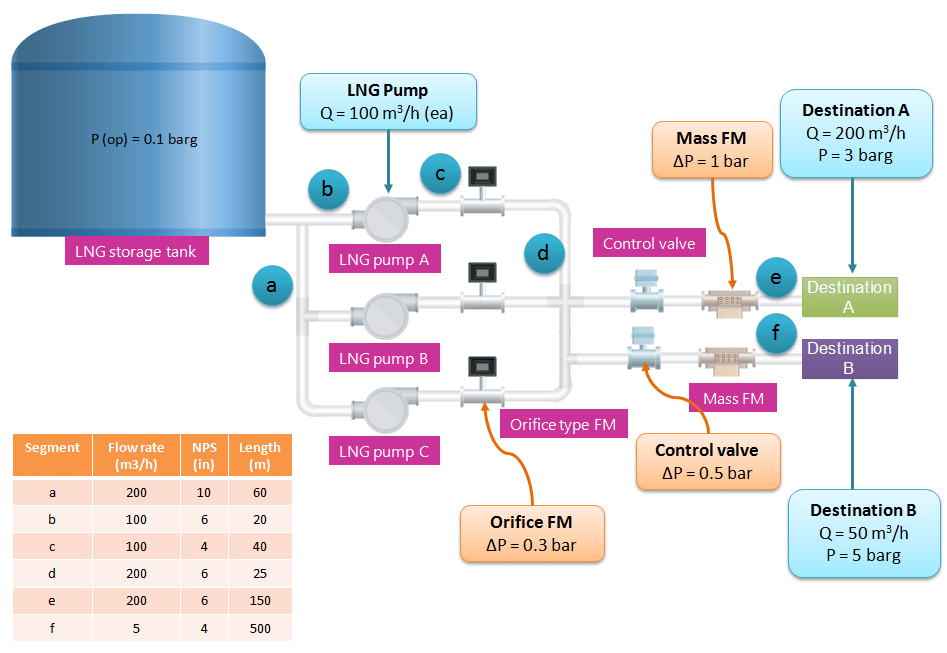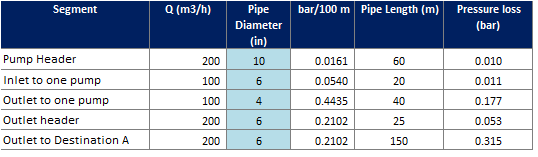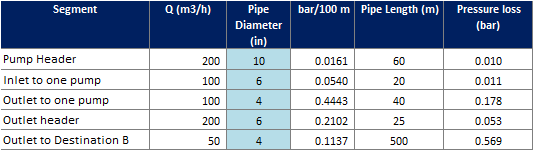Skip to main content# How to Properly Size Discharge Pressure of Pump

When sizing discharge pressure of pump, we need to consider at least two things: target pressure at destination point and pressure loss from source point to destination point. How if we have two different destination points? How do we properly size pump discharge pressure?

In this post I want to share you simple method to size discharge pressure of pump when you have more than one destination point.

Let say we have a system to transport LNG from LNG flat bottom storage tank to two different destination points at different rate. Rate of LNG at Destination A is 200 m3/h with pressure at its destination is 3 barg. While LNG at Destination B is 50 m3/h with pressure  barg.illustration-for-pressure-loss-calculation

To transport the LNG we have 3 x 50% pump. Capacity of each pump is 100 m3/h. So, to meet LNG requirement at pump A we have to operate two pumps simultaneously.

In this system, I have assumption of length and pipe diameter of each pipe section. Please see the picture.

First of all, to determine required discharge pressure of pump, you need to consider pressure loss. Sources of pressure loss are pipes, equipments, fittings, valve, and other components installed in the pipe, such as flow element. Look at the system and we can conclude that sources of pressure loss are:

• Pipe
• Orifice flow meter
• Control valve
• Mass flow meter

I have assumed pressure loss on each piping components. Please see picture above.

Now, we start to calculate required pressure drop for the case of pumping LNG to Destination A. I calculate pressure loss due to pipe in each segment.

Picture below show the result of pressure loss due to piping to Destination A.pipe-pressure-loss-at-destination-a

Total piping pressure loss is 0.6 barg. Then we calculate pressure due to other piping components. Grand total pressure loss due to piping and piping components is 2.37 barg. Because required pressure at Destination A is 3 barg, then we add pressure loss and required pressure. We get 5.36 barg. This value is minimum pump discharge pressure when transporting LNG to Destination A.pressure-loss-component-at-destination-a

How about differential head? Because pressure at tank is 0.1 barg, then differential pressure is 5.27 barg, which is equal to 116.8 m (assuming LNG density 460 kg/m3).

Then, we look into Destination B. We use the same method as we applied in calculating discharge pressure at Destination A. We get total pressure loss is 2.62 barg, slightly higher than that in Destination A. Thus minimum required discharge pressure is 7.62 barg. Differential pressure is 7.52 barg which is equal to 166.8 m.pipe-pressure-loss-at-destination-bpressure-loss-component-at-destination-b

We compare result we get at Destination A and Destination B. We see that required pressure at Destination B is higher than that of Destination A. So, we conclude that we need to select the highest differential pressure among those two. We choose 166.8 m differential pressure.

Hopefully this post is simple enough to give you clear understanding about how to size discharge pressure of pump.